# 15 multiple choice calcAnonymous
timer Asked: Mar 3rd, 2019
account_balance_wallet \$15

### Question Description

1.

Evaluate the integral: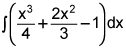(4 points)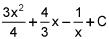x4 + 2x3 - x + C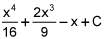2.

Evaluate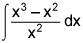. (4 points)

 x - 1 + C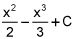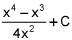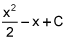3.

Evaluate the integral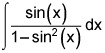. (4 points)

 2ln|1 - sin2(x)| + C -csc(x) + C sec(x) + C None of these

4.

If f(x) and g(x) are continuous on [a, b], which one of the following statements is true? (4 points)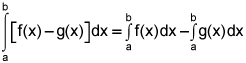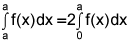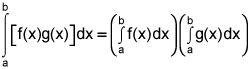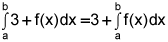5.

Evaluate the integral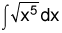. (4 points)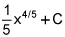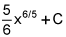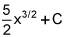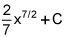1.

Evaluate the integral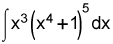. (4 points)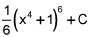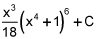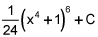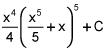2.

Evaluate the integral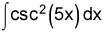. (4 points)

 -2csc(5x)cot(5x) + C -10csc(5x)cot(5x) + C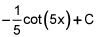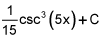3.

Which of the following integrals cannot be evaluated using a simple substitution? (4 points)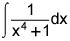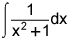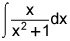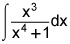4.

Evaluate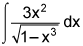. (4 points)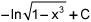-ln|1 - x3| + C sin-1(x3) + C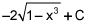5.

Evaluate the integral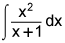. (4 points)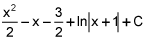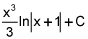x - 1 + ln|x| + C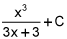1.

Which of the following definite integrals could be used to calculate the total area bounded by the graph of y = 1 - x2 and the x-axis? (4 points)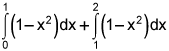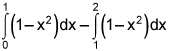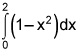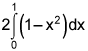2.

Suppose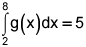, and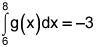, find the value of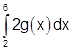. (4 points)

 8 12 16 4

3.

Evaluate the integral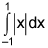. (4 points)

 1 0 2 -1

4.

Use your graphing calculator to evaluate to three decimal places the value of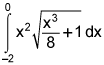. (4 points)

 0.25 2.667 1.778 -3.251

5.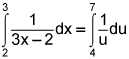(4 points)

 True False

School: Rice Universityflag Report DMCAReviewAnonymous
awesome work thanksBrown University

1271 TutorsCalifornia Institute of Technology

2131 TutorsCarnegie Mellon University

982 TutorsColumbia University

1256 TutorsDartmouth University

2113 TutorsEmory University

2279 TutorsHarvard University

599 TutorsMassachusetts Institute of Technology

2319 TutorsNew York University

1645 TutorsNotre Dam University

1911 TutorsOklahoma University

2122 TutorsPennsylvania State University

932 TutorsPrinceton University

1211 TutorsStanford University

983 TutorsUniversity of California

1282 TutorsOxford University

123 TutorsYale University

2325 Tutors